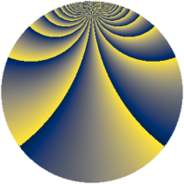# Properties

 Label 1323.2.bmLevel $1323$ Weight $2$ Character orbit 1323.bm Rep. character $\chi_{1323}(64,\cdot)$ Character field $\Q(\zeta_{21})$ Dimension $648$ Sturm bound $336$

# Related objects

## Defining parameters

 Level: $$N$$ $$=$$ $$1323 = 3^{3} \cdot 7^{2}$$ Weight: $$k$$ $$=$$ $$2$$ Character orbit: $$[\chi]$$ $$=$$ 1323.bm (of order $$21$$ and degree $$12$$) Character conductor: $$\operatorname{cond}(\chi)$$ $$=$$ $$441$$ Character field: $$\Q(\zeta_{21})$$ Sturm bound: $$336$$

## Dimensions

The following table gives the dimensions of various subspaces of $$M_{2}(1323, [\chi])$$.

Total New Old
Modular forms 2088 696 1392
Cusp forms 1944 648 1296
Eisenstein series 144 48 96

## Trace form

 $$648q + 5q^{2} + 47q^{4} + 9q^{5} - 7q^{7} + 28q^{8} + O(q^{10})$$ $$648q + 5q^{2} + 47q^{4} + 9q^{5} - 7q^{7} + 28q^{8} - 28q^{10} + 5q^{11} - 7q^{13} + 38q^{14} + 47q^{16} + 16q^{17} - 44q^{19} + 29q^{20} - 13q^{22} + 20q^{23} + 41q^{25} + 20q^{26} - 28q^{28} + 35q^{29} - 20q^{31} + 25q^{32} - q^{34} + 44q^{35} - 30q^{37} + 7q^{38} + 5q^{40} + 29q^{41} - 13q^{43} + 88q^{44} + 32q^{46} + 55q^{47} - q^{49} - 6q^{50} + 3q^{52} + 136q^{53} - 100q^{55} - 145q^{56} + 17q^{58} + 19q^{59} + 42q^{61} - 96q^{62} - 124q^{64} + 11q^{65} - 26q^{67} - 166q^{68} - 7q^{70} + 22q^{71} + 8q^{73} + 45q^{74} - 41q^{76} - q^{77} - 26q^{79} + 440q^{80} - 28q^{82} - 61q^{83} + 5q^{85} - 15q^{86} - q^{88} - 22q^{89} - 16q^{91} + 43q^{92} - q^{94} + 38q^{95} - 14q^{97} + 144q^{98} + O(q^{100})$$

## Decomposition of $$S_{2}^{\mathrm{new}}(1323, [\chi])$$ into newform subspaces

The newforms in this space have not yet been added to the LMFDB.

## Decomposition of $$S_{2}^{\mathrm{old}}(1323, [\chi])$$ into lower level spaces

$$S_{2}^{\mathrm{old}}(1323, [\chi]) \cong$$ $$S_{2}^{\mathrm{new}}(441, [\chi])$$$$^{\oplus 2}$$